• matlab中mod和rem的区别
2021-04-24 20:07:27

通常取模运算也叫取余运算，它们返回结果都是余数.rem和mod唯一的区别在于:

当x和y的正负号一样的时候，两个函数结果是等同的；当x和y的符号不同时，rem函数结果的符号和x的一样，而mod和y一样。

这是由于这两个函数的生成机制不同，rem函数采用fix函数，而mod函数采用了floor函数(这两个函数是用来取整的，fix函数向0方向舍入，floor函数向无穷小方向舍入)。

rem(x，y)命令返回的是x-n.*y，如果y不等于0，其中的n = fix(x./y)，而mod(x,y)返回的是x-n.*y，当y不等于0时，n=floor(x./y)

两个异号整数取模取值规律 (当是小数时也是这个运算规律，这一点好像与C语言的不太一样)

先将两个整数看作是正数，再作除法运算

①能整除时，其值为0

②不能整除时，其值=除数×(整商+1)-被除数

例：mod(36,-10)=-4

即：36除以10的整数商为3，加1后为4；其与除数之积为40；再与被数之差为(40-36=4)；取除数的符号。所以值为-4。

例：mod(9,1.2)=0.6;

例：

>> mod(5,2)

ans =1                   %“除数”是正，“余数”就是正

>> mod(-5,2)

ans =1

>> mod(5,-2)

ans =-1                  %“除数”是负，“余数‘就是负

>> mod(-5,-2)

ans =-1                  %用rem时，不管“除数”是正是负，“余数”的符号与“被除数”的符号相同

>> rem(5,2)

ans =1                   %“被除数”是正，“余数”就是正

>> rem(5,-2);

ans =1

>> rem(-5,2)

ans =-1                 %“被除数”是负，“余数”就是负

>> rem(-5,-2)

ans =-1

本文来源于网络：查看 >https://blog.csdn.net/u012939857/article/details/64141356

更多相关内容
•MATLAB
• 如果xy的符号相同（同为‘+’，同为‘-’），那么rem(x,y)=mod(x,y)（正数与正数，负数与负数，取整结果两个函数效果一样） 如果xy的符号相反，那么mod(x,y)=rem(x,y)+y(正数与负数的取整，看你希望得到...
%求整数x/y的余数
rem(x,y)
%求模
mod(x,y)

如果x和y的符号相同（同为‘+’，同为‘-’），那么rem(x,y)=mod(x,y)（正数与正数，负数与负数，取整结果两个函数效果一样）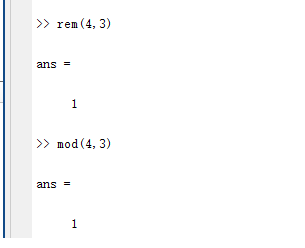如果x和y的符号相反，那么mod(x,y)=rem(x,y)+y(正数与负数的取整，看你希望得到什么结果就选择用什么函数)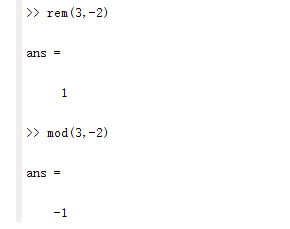当正数与负数取余时，当得到的余数结果的符号希望跟除数(x)一样，用rem()函数；当得到的余数结果的符号希望跟被除数(y)一样，用mod()函数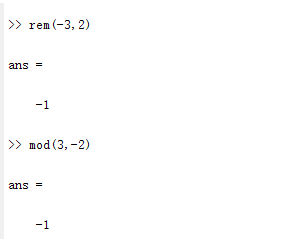展开全文matlab
•   求余函数求模函数有相同的地方但又不完全一致，主要的区别在于对负整数进行...  remmod函数的区别是，当b≠0b\ne 0b​=0时，rem(a,b)=a-b.*fix(a./b)，而mod(a,b)=a-b.*floor(a./b);当b=0b=0b=0时，rem(a,

求余函数和求模函数有相同的地方但又不完全一致，主要的区别在于对负整数进行除法运算的操作不同。对于整数a，b来说，求余运算或求模运算的方法都是先求整数商c=a/b，再求余数或模r=a-c*b。求余运算在取c的值时，向0方向取整（fix函数），而求模运算在计算c的值时，向负无穷方向取整（floor函数）。
rem与mod函数的区别是，当 b ≠ 0 b\ne 0 时，rem(a,b)=a-b.*fix(a./b)，而mod(a,b)=a-b.*floor(a./b);当 b = 0 b=0 时，rem(a,0)=NaN，而mod(a,0)=a。
如果a、b符号相同，那么rem(a,b)=mod(a,b)。如果a、b符号相反，那么mod(a,b)=rem(a,b)+b。rem(a,b)的符号与a相同，而mod(a,b)的符号与b相同。例如，rem(7,4)=mod(7,4)=3；rem(-7,-4)=mod(-7,-4)=-3；rem(7,-4)=3，而mod(7,-4)=-1。

当正数与负数取余时，当得到的余数结果的符号希望跟除数(x)一样，用mod()函数；当得到的余数结果的符号希望跟被除数(y)一样，用rem()函数

展开全文matlab
• rem mod 区别

# 取余(rem)和取模(mod)的区别

Matlab

生成机制
取余：采取fix()函数，向0方向取整
取模：采取floor()函数，向无穷小方向取整
当A，B异号时（其实同号也是这个规律）
取余：结果和A同号
取模：结果和B同号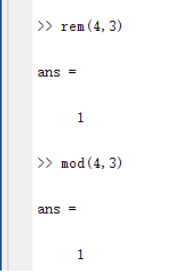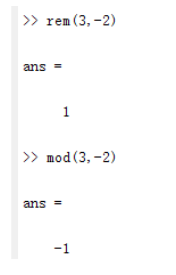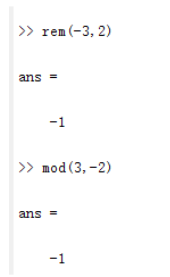PS：“%”在js、c/c++、java 中为" 取余 “, Python 中是” 取模 "

展开全文matlab
• rem和mod唯一的区别在于: 当xy的正负号一样的时候，两个函数结果是等同的；当xy的符号不同时，rem函数结果的符号x的一样，而mod和y一样。 这是由于这两个函数的生成机制不同，rem函数采用fix函数，而mod函数...
• mod函数用于取模运算。 语法形式为 M = mod(x,y)，这里的x,y可以是标量|向量|矩阵|多维数组。 M = mod(x,y)返回用 y除以 x后的余数，其中 x是被除数，y是除数。 计算方式为：当 y≠0 时，M=mod(x,y)= x - y.*...
• M=mod(X,Y):M = mod(X,Y) if Y ~= 0, returns X - n.*Y where n = floor(X./Y). If Y is not an integer and the quotient X./Y is within roundoff error of an integer, then n is that integer. The inputs X and...
• rem mod （除后的余数） 语法： r=rem(a,b) ; b=mod(a,m) 其中 a 是被除数： 被除数，指定为标量、向量、矩阵或多维数组。a 必须是任意数值类型的实数值数组。数值输入 a b 必须具有相同的大小或兼容的大小...matlab
• rem(x,y):求整除x/y的余数mod(x... (floor()向左取整)如果xy的符号相同(同为‘+’，同为‘-’)，那么rem(x,y)=mod(x,y)(正数与正数，负数与负数，取整结果两个函数效果一样)如果xy的符号相反，那么mod(x,y)=rem(...
• rem(x,y):求整除x/y的余数mod(x... (floor()向左取整)如果xy的符号相同(同为‘+’，同为‘-’)，那么rem(x,y)=mod(x,y)(正数与正数，负数与负数，取整结果两个函数效果一样)如果xy的符号相反，那么mod(x,y)=rem(...
• 学习Matlab的数学函数时，教程提到取模（mod）与取余（rem）是不同的matlab
• 满意答案libaoxin2008推荐于 2019.10.24matlabrem()和mod()函数(2010-11-16 13:39:55) 转载rem(x,y):求整除x/y的余数mod(x,y):求模rem(x,y)=x-y.*fix(x./y); (fix()向0取整)mod(x,y)=x-y.*floor(x./y); (floor()向...
• rem和mod是求余的，但是算法不同，差异在哪呢？   就在于分别使用了fixfloor, 函数fixfloor都是圆整用的，fix是向0的方向圆整，如fix(-1.5)=-1,fix(1.5)=1;而floor是向下圆整，如floor(-1.5)=-2,floor(1.5...MATLAB
• matlab——fix，floor，ceil，round区别总结为：fix朝零方向取整，floor朝负无穷方向取整，ceil朝正无穷方向取整，round四舍五入到最近的整数(1)fix(x):截尾取整。如：>> fix([3.4 , -3.4])ans =3 -3(2)floor...
• An example of rem and modmatlab MOD
• (floor()向左取整,以数抽为准，朝负无穷方向取整)如果xy的符号相同(同为‘+’，同为‘-’)，那么rem(x,y)=mod(x,y)(正数与正数，负数与负数，取整结果两个函数效果一样)如果xy的符号相反，...
• 通常取模运算也叫取余运算，它们返回结果都是余数.rem和mod唯一的区别在于:  当xy的正负号一样的时候，两个函数结果是等同的；当xy的符号不同时，rem函数结果的符号x的一样，而mod和y一样。  这是由于这两个...
• ## matlab中rem与mod函数的区别

万次阅读 多人点赞 2015-10-10 08:50:53
语法格式： rem(x,y):求整除x/y的余数 mod(x,y):求模 ...rem(x,y)=x-y.*fix(x./y);...mod(x,y)=x-y.*floor(x./y);...如果xy的符号相同（同为‘+’，同为‘-’），那么rem(x,y)=mod(x,y)（正数与正数matlab rem
• MOdModulusafter divisionSyntax 语法M = mod(X,Y)DescriptionM =mod(X,Y)ifY ~= 0,returnsX -n.*Ywheren =floor(X./Y).IfYis not an integerand the quotientX./Yiswithin roundoff error of an integer,the...
• 昨天在学习Matlab的数学函数时，教程提到取模(mod)与取余(rem)是不同的，今天在网上具体查了一下：通常取模运算也叫取余运算，它们返回结果都是余数.rem和mod唯一的区别在于:当xy的正负号一样的时候，两个函数...
• mod的结果取决于除数的符号，如果除数是正，则余数也为正；反之则余数为负。 mod(1,4)=1; mod(-2,3)=1; mod(2,-3)=-1; rem的结果取决于被除数的符号，如果被除数为正，则余数也为正；反之则余数为负。 rem(1,4)=1; ...
• 分别看两个官方说明，加了一些中文： 一些例子：Matlab
• Matlab中取余运算rem和取模运算mod区别及用法matlab...